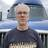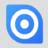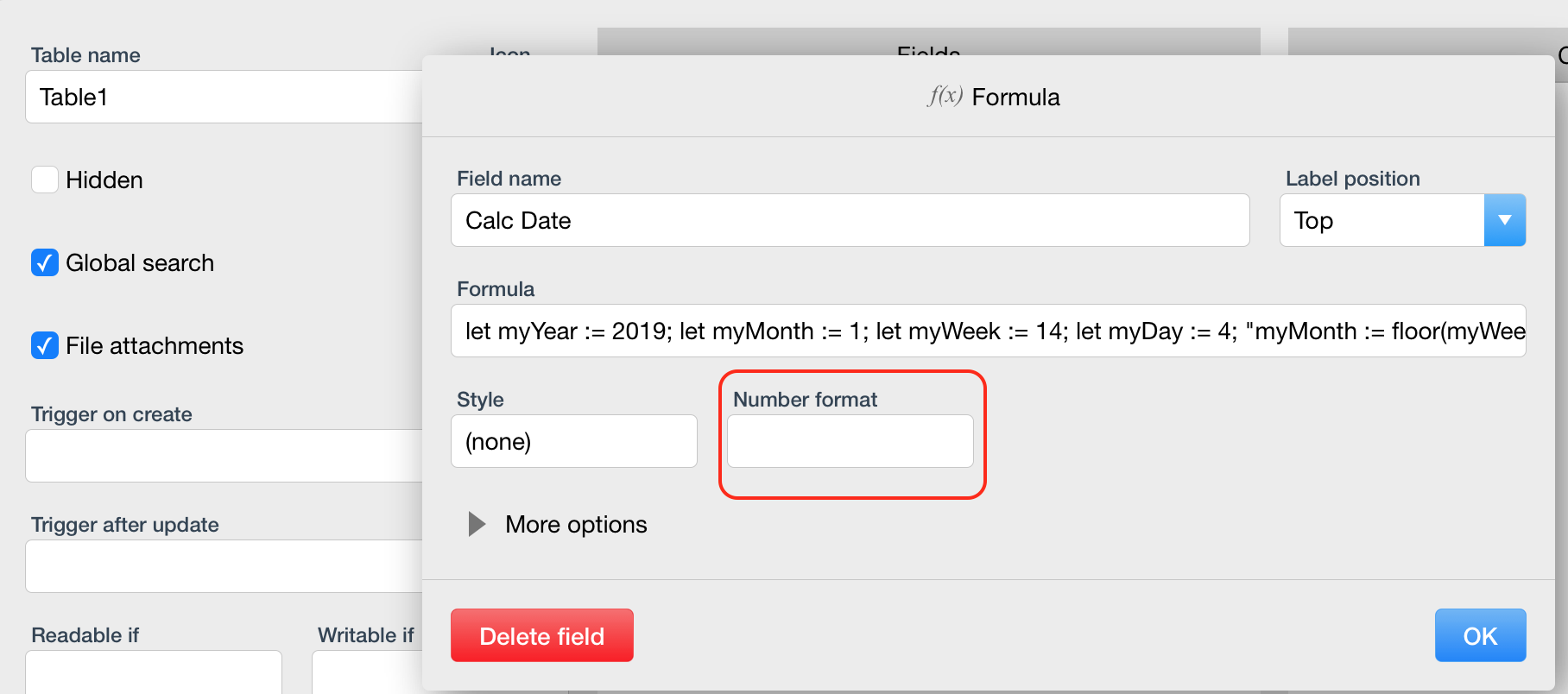Calculate a future date

Am a new user, have developed a database for vehicle service. I want to calculate months (6, 12, 18, 36) from the completed date that the next inspection is due.

Am using: if 'Service Type' = 4 and 'Miles or Months' = 2 and 'Item' = 5 then (completed date) + (months)

else null;

end

This works, but returned 1560582000000https://ninoxdb.de/en/manual/calculations/reference-of-functions-and-language

Try using the date() function. The result was 06/15/2019 for me.

Entered this strig:

if 'Service Type' = 4 and 'Miles or Months' = 2 and Item = 8 then
today() + 365
else

it works, but returns a very large number. How can I change it to show a future date? The string should display 1 year from now?Hi,

`... then date(today() + 365)`

`else....`

Best, Jörg

Thank you. I tried that but it returns a huge number in the field. At first I thought it was the number of seconds in a year, but it is much larger than 31,557,600.

Surely im doing something wrong!Hi,

Where did you enter the script? In a formula field?

Is that the complete script?

Kind regards, Jörg

Yes sir, it is a formula field.

I have a database to track vehicle maintenance. Part of the information is inspection or replacement. Some requirements are based on Months, others on Miles.This is the string to determine next Due date:

if 'Service Type' = 4 and 'Miles or Months' = 1 and Item = 1 then

'Current Mileage' + 3500

else

if 'Service Type' = 4 and 'Miles or Months' = 1 and Item = 2 then

'Current Mileage' + 3500

else

if 'Service Type' = 4 and 'Miles or Months' = 1 and Item = 3 then

'Current Mileage' + 5000

else

if 'Service Type' = 4 and 'Miles or Months' = 1 and Item = 4 then

'Current Mileage' + 75000

else

if 'Service Type' = 4 and 'Miles or Months' = 2 and Item = 5 then

date(today() + 365)

else

if 'Service Type' = 4 and 'Miles or Months' = 2 and Item = 8 then

date(today() + 365)

else

if 'Service Type' = 4 and 'Miles or Months' = 2 and Item = 7 then

date(today() + 1080)

else

if 'Service Type' = 4 and 'Miles or Months' = 2 and Item = 6 then

date(today() + 1080)

else

null

end

end

end

end

end

end

end

end

The code works fine for miles, but for months, it returns a huge number.Make sure Number format is empty...Yes sir, it is empty.

I see your formula is different - is that for your test?

1 2